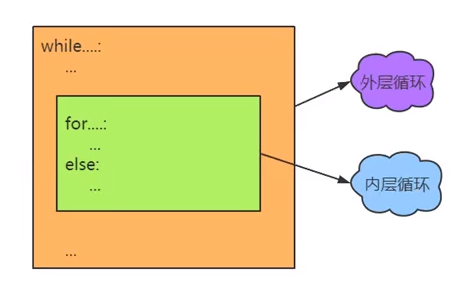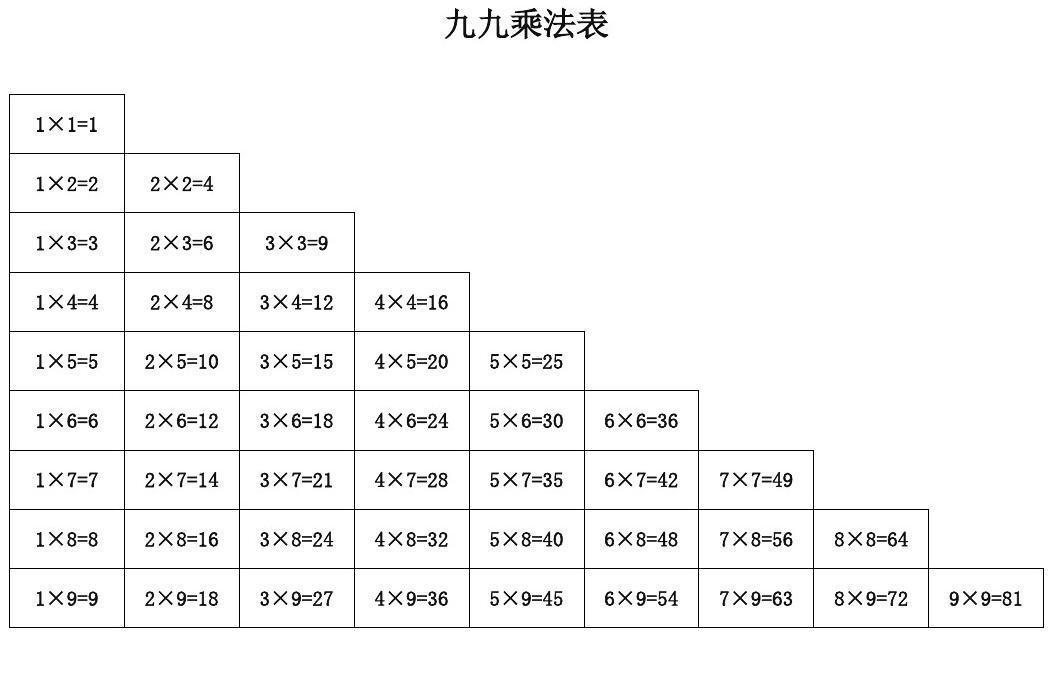for...in...嵌套循环做个简单的演示（三行四列打印输入*

for _ in range(3):
for _ in range(4):
print('*', end='\t')
print()

• *---*---*---*---
• *---*---*---*---
• *---*---*---*---for a in range(1, 10):
for b in range(1, a + 1):
print('*', end='\t')
print()

• *---
• *---*---
• *---*---*---
• *---*---*---*---
• *---*---*---*---*---
• *---*---*---*---*---*---
• *---*---*---*---*---*---*---
• *---*---*---*---*---*---*---*---
• *---*---*---*---*---*---*---*---*---

for a in range(1, 10):
for b in range(1, a + 1):
print(b, '*', a, '=', b * a, end='\t')
print()

• 1 * 1 = 1---
• 1 * 2 = 2---2 * 2 = 4---
• 1 * 3 = 3---2 * 3 = 6---3 * 3 = 9---
• 1 * 4 = 4---2 * 4 = 8---3 * 4 = 12---4 * 4 = 16---
• 1 * 5 = 5---2 * 5 = 10---3 * 5 = 15---4 * 5 = 20---5 * 5 = 25---
• 1 * 6 = 6---2 * 6 = 12---3 * 6 = 18---4 * 6 = 24---5 * 6 = 30---6 * 6 = 36---
• 1 * 7 = 7---2 * 7 = 14---3 * 7 = 21---4 * 7 = 28---5 * 7 = 35---6 * 7 = 42---7 * 7 = 49---
• 1 * 8 = 8---2 * 8 = 16---3 * 8 = 24---4 * 8 = 32---5 * 8 = 40---6 * 8 = 48---7 * 8 = 56---8 * 8 = 64---
• 1 * 9 = 9---2 * 9 = 18---3 * 9 = 27---4 * 9 = 36---5 * 9 = 45---6 * 9 = 54---7 * 9 = 63---8 * 9 = 72---9 * 9 = 81---